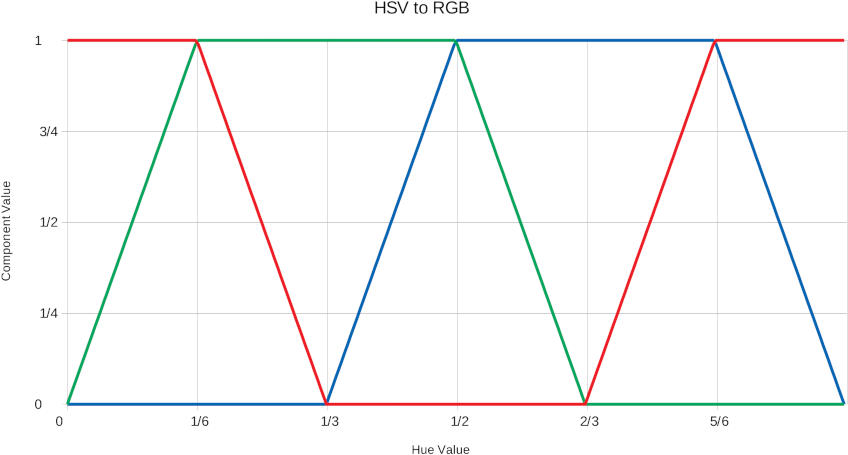Categories

# HSV(ish) Colour Wheel in Python

Years back I wrote something about HSV colour cycling for Arduino. Things have moved on: we’re all writing code in MicroPython/CircuitPython now and 8-bit micro-controllers are looking decidedly quaint. Yes, yes; some of you must still write code in PIC assembly language and I’m sure that’s very lovely for you indeed don’t @ me.

If you look at the output of a typical HSV to RGB algorithm, the components map something like this:Hue between 0-1, with saturation and value set to 1. Output range 0-1 for each component

These lines remind me so much of sine waves, if very blocky ones. The red trace in particular is just the cosine function, with the input range of 0..2π and the output range of -1..1 both mapped to 0..1. The green and blue traces are just the red trace shifted horizontally by ⅓ and ⅔ respectively.

Since we have transcendental functions in MicroPython, we don’t have to fossick about with linear approximations. The common RGB LED function wheel() uses similar linear interpolation as the graph above. Why make do with blocky cogwheels when you can have a smooth colour wheel?

```def cos_wheel(pos):
# Input a value 0 to 255 to get a colour value.
# scruss (Stewart Russell) - 2019-03 - CC-BY-SA
from math import cos, pi
if pos < 0:
return (0, 0, 0)
pos %= 256
pos /= 255.0
return (int(255 * (1 + cos( pos            * 2 * pi)) / 2),
int(255 * (1 + cos((pos - 1 / 3.0) * 2 * pi)) / 2),
int(255 * (1 + cos((pos - 2 / 3.0) * 2 * pi)) / 2))
```